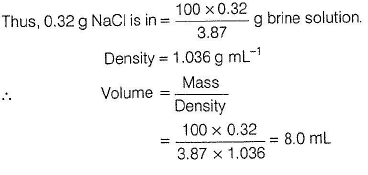Courses

# Test: Different Methods Of Expressing Concentration Of Solutions

## 30 Questions MCQ Test Chemistry Class 12 | Test: Different Methods Of Expressing Concentration Of Solutions

Description
This mock test of Test: Different Methods Of Expressing Concentration Of Solutions for NEET helps you for every NEET entrance exam. This contains 30 Multiple Choice Questions for NEET Test: Different Methods Of Expressing Concentration Of Solutions (mcq) to study with solutions a complete question bank. The solved questions answers in this Test: Different Methods Of Expressing Concentration Of Solutions quiz give you a good mix of easy questions and tough questions. NEET students definitely take this Test: Different Methods Of Expressing Concentration Of Solutions exercise for a better result in the exam. You can find other Test: Different Methods Of Expressing Concentration Of Solutions extra questions, long questions & short questions for NEET on EduRev as well by searching above.
QUESTION: 1

### Only One Option Correct Type This section contains 10 multiple choice questions. Each question has four choices (a), (b), (c) and (d), out of which ONLY ONE is correct Which of the following concentration factors is affected by change in temperature?

Solution:

On changing temperature, solvent (H2O) is vaporised or condensed. Thus, volume of solution and solvent changes. Thus, any concentration factor dependent on volume is affected by change in temperature.
(a) Molarity = Mole/Litre (Volume dependent)
(b) Molality = Moles of solute/kg of solvent (weight dependent)
(c) Mole fraction = ni/ntotal(unitless)
(d) Weight fraction = wi/wtotal (unitless)

QUESTION: 2

### 6.02 x1020 molecules of urea are present in 100 mL of its solution. The concentration of urea solution is (N0 = 6.02 x 1023 mol-1) [AIEEE 2004]

Solution: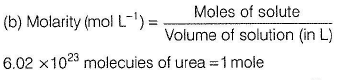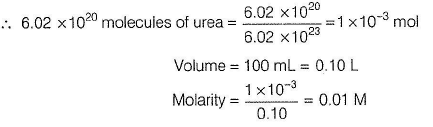QUESTION: 3

### Glucose solution is one molal. Glucose present in 1 kg glucose solution is

Solution:

Glucose solution is one molal. Thus, 1000 g water (solvent) has glucose = one mole = 180 g

Total mass of solution = 1000 +180 = 1180 g

1180 g of solution has glucose = 180 g

∴ 1000 g of solution has glucose =
180 x 1000/1180 = 152.5 ≈ 153g

QUESTION: 4

The molality of a urea solution in which 0.0100 g of urea (NH2CONH2) is added to 0.300 dm3 of water at STP is

[AIEEE 2011]

Solution: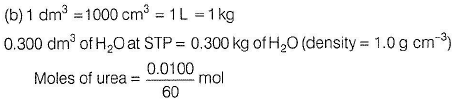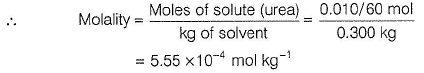QUESTION: 5

A solution that is 20% ethanol by volume is found to have a density of 0.977 g/mL. Density of ethanol is 0.789 g/mL. Thus, mass per cent of ethanol solution is

Solution:

20% ethanol by volume means 100 m l of (ethanol + H20 ) solution has ethanol = 20 mL 100 mL of solution = 100 x 0.977 g solution = 97.7 g solution 20 mL ethanol = 20 x 0.789 g ethanol = 15.78 g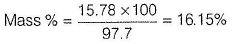QUESTION: 6

A solution of NH4CI is prepared by dissolving 95 g of NH4CI in 200 g of H2O at 60° C. What is mass per cent when the solution is cooled to 20° C based on this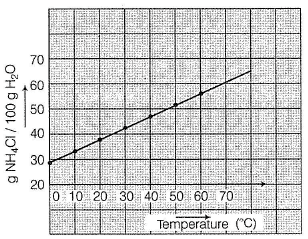Solution:

200 g H2O at 60°C has 95 g NH4Cl

Based on solubility graph, at 20°C,

100 g of H2O can dissolve = 36 g NH4CI

hence, 200 g of H20 can dissolve = 72g  NH4CI

Thus, NH4CI separated (crystallised) = 95 - 72 = 23 g

Mass % = 36%

QUESTION: 7

Commercial concentrated nitric acid is 15.6 M. To prepare 10 L of 6.0 M nitric acid from it,

Solution:

This is a case of dilution.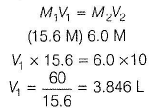Thus, 3.846 L of 15.6 M solution is diluted to 10.0 L by addition of 6.154 L of H20.

QUESTION: 8

AgNO3 sample is 85% by mass. To prepare 125 mL of 0.05 M AgNO3 solution, AgNO3 sample required is

Solution:

125 mL of 0.05 M AgN03 = 125 x 0.05 millimoles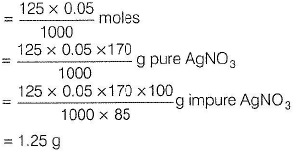QUESTION: 9

Mole fraction of C3H5(OH)3 in a solution of 36 gm of water and 46 gm of glycerine is

Solution:

The correct answer is option C
The molar mass M2​ of non-volatile substance can be calculated from the following formula.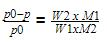Here, p0 vapour pressure of acetone and p is the vapour pressure of the solution.
M1​ and W1​ are the molar mass and mass of acetone.
M2​ and W2​ are the molar mass and mass of a non-volatile substance.
Substitute values in the above expression.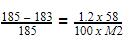Hence, M2​=64.38g/mol.
Thus, the molar mass of the non-volatile substance is 64 g/mol (approximately).

QUESTION: 10

Hardness of a water sample is 200 ppm CaCO3, Thus, molarity of CaCO3 is

Solution:

Hard water has hardness = 200 ppm Thus, 106 mL(= 103 L)water has CaC03 =200 g =2 mol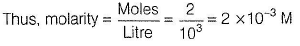QUESTION: 11

100 mL of aqueous solution of 0.01 M CaCI2 is evaporated to dryness when 0.15 g of residue is obtained. Thus, impurity present is

Solution:

100mLof 0.01 M CaCI2 = 100 x 0.01 millimoles CaCI2
= 0.001 mole CaCI2
= 0.001 x 111 g CaCI2
= 0.111 g pure CaCI2
But, residue = 0.15 g
Thus, impurity = 0.15 - 0.111= 0.039 g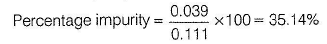QUESTION: 12

At 25 ° C, the density of 18 MH2SO4 is 1.8 g cm3. Thus, mass percentage of H2SO4 in aqueous solution is

Solution:

18 MH2SO4 means18 mol L-1.
1000 mL of H2S04 solution has H2SO4 = 18 mol
= 18 x98g
Also, 1000 m L = 1000 x 1.8 g H2SO4 solution
Thus, (1000 x 1.8)g H2SO4 solution has H2SO4 =18 x 98 g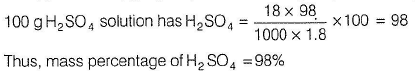QUESTION: 13

Laboratory ammonia is 14.8 M NH3(ag)with a density of 0.8980 g/mL. Mole fraction of NH3 in this solution is

Solution:

14.8 M NH3(ag ) means 14.8 moles NH3 in 1000 mL solution . 1000 mL solution = 1000 x 0,898 g solution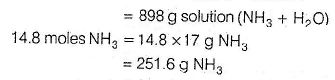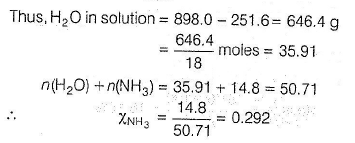QUESTION: 14

An aqueous solution is 34% H3P04 by mass and has density 1.209 g mL -1 Molarity (I), molality (II) and normality (III) respectively, are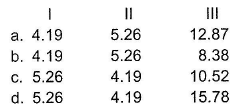Solution:

100g H3P04 aqueous solution has 34g H3P04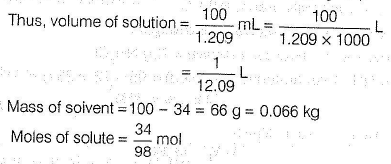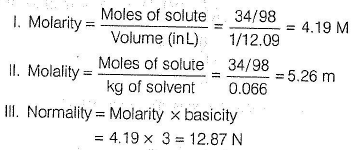QUESTION: 15

If one assumes the volumes are additive, what is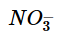in a solution obtained by mixing 275 mL of 0.200 M KN03, 325 mL of 0.40 M Mg(N03)2 and 400 mL H20?

Solution: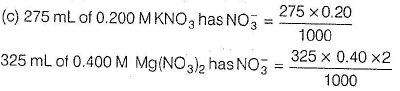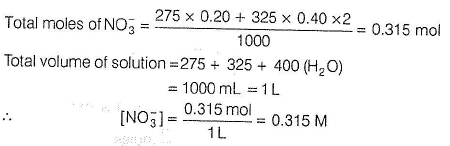QUESTION: 16

How many grams of water would you add to 1.38 moles of CH3OH in 1 kg water to reduce the molality to 1.00 molal CH3OH(aq) ?

Solution:

1.38 moles of CH3OH in w kg water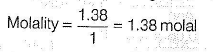1.38 moles of CH3OH in w Kg water

So that molarity = 1.0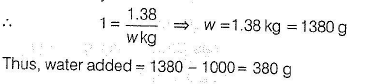QUESTION: 17

Three solutions have been provided.

I. 3 g HCI in 1 kg H20.
II. 2 L HCI(g) and 1 L H20 at room temperature.
III. Aqueous solution of HCI with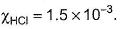Solutions containing same number of moles of HCI is/are

Solution: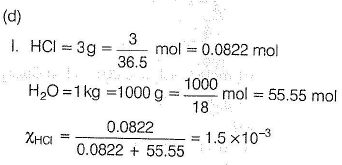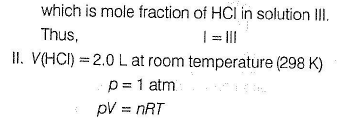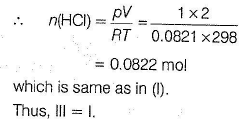QUESTION: 18

Which of the following aqueous solutions has the highest concentration of Na+?

Solution: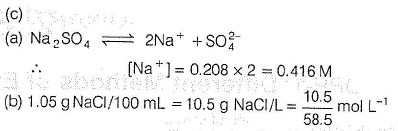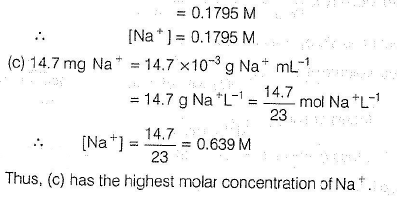QUESTION: 19

pH of Ba(OH)2 solution is 12. Number of millimoles present in 100 mL of Ba(OH)2 solution is

Solution: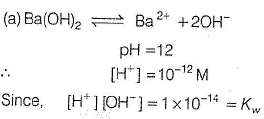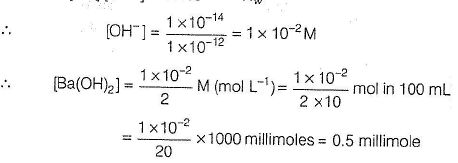QUESTION: 20

We have
I. 25 mL of 1 M NaOH
II. 10 mL of 0.50 M NaCI
On mixing the two solutions, molar concentrations of Na+, OH- and Cl- respectively, are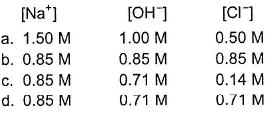Solution:

This is a case of dilution.
NaOH solution has been diluted by NaCI or vice-versa.
Total volume = 35.0 mL

M1V1 = M2V2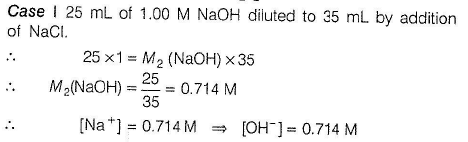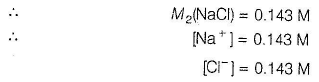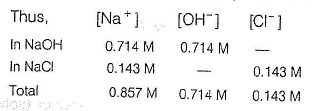QUESTION: 21

A ‘100 proof solution of ethanol in water consists of 50.0 mL of C2H5OH(/)and 50.0 mL of H20 (/), mixed at 16 °C . Given,
Density of H20 = 1 g mL-1
Density of C2H,OH = 0.7939 gmL-1
Density mixture = 0.9344 g mL-1
Volume of the solution is

Solution:

Volume can be additive or not depend on the nature of the solution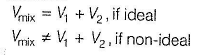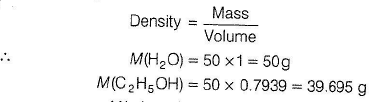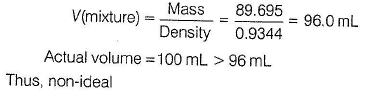QUESTION: 22

How many grams of Mgl2 must be added to 250 mL of 0.0876 M Kl to produce a solution with [l-] = 0.10 M?

Solution: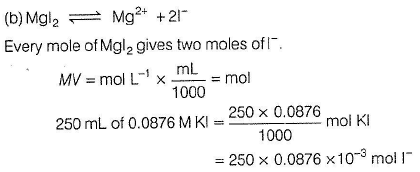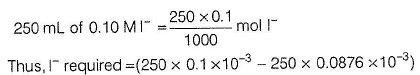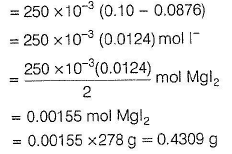QUESTION: 23

An aqueous solution is 6% methanol, CH3OH, by mass with d = 0.988 g mL-1. Thus, molarity of CH3OH in this solution is

Solution:

(d) Aqueous 6% methanol solution by mass means 100 g of aqueous solution has = 6g CH3OH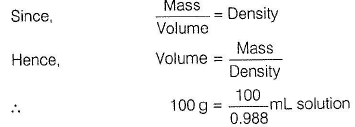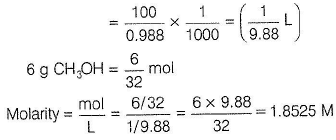QUESTION: 24

Matching List Type
Choices for the correct combination of elements from Column I and Column II are given as options (a), (b), (c) and (d), out of which one is correct

An ethanol-water solution is prepared by dissolving 10.00 mL o f ethanol (C2H5OH, d = 0.789 g mL-1) in a sufficient volume o f w ater to produce 100 mL of solution with a density, d = 0.982 g mL-1. Match the concentration term in Column I with its value in Column II and select the answer from the code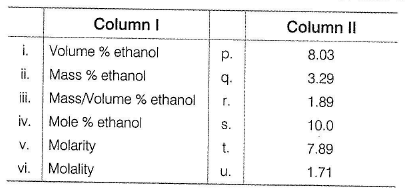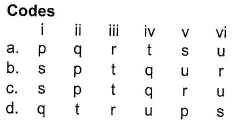Solution: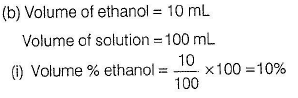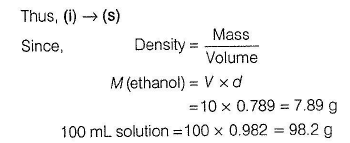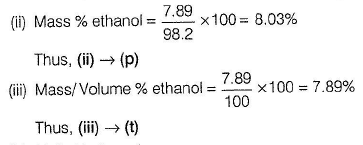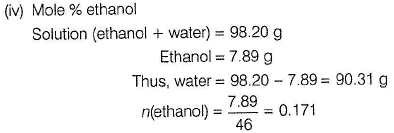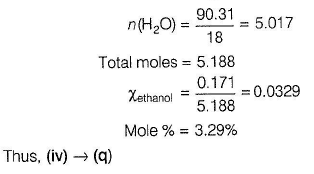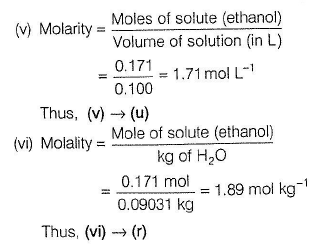*Multiple options can be correct
QUESTION: 25

One or More than One Options Correct Type
This section contains 2 multiple type questions. Each question has 4 choices (a), (b), (c) and (d), out of which ONE or MORE THAN ONE are correct.

1 kg of aqueous solution has 0.40 kg NaOH. Thus, this solution is

Solution:

1 kg aqueous solution = 1000 g aqueous solution

It has 0.4 kg NaOH = 0.4 x1000= 400 g NaOH

Thus, solvent (water) =1000 - 400 = 600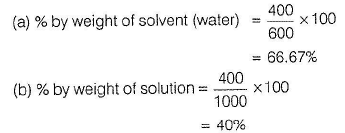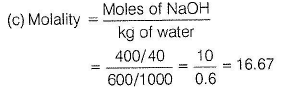(d) Since, density is not given hence, molarity cannot be derive

*Multiple options can be correct
QUESTION: 26

Mole fraction of ethanol in ethanol-water solution is 0.25. Thus, this solution is

Solution: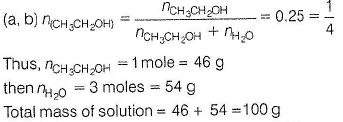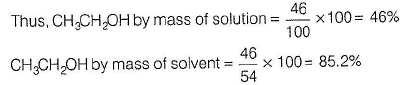QUESTION: 27

Comprehension Type

This section contains a passage describing theory, experiments, data, etc. Two questions related to the paragraph have been given. Each question has only one correct answer out of the given 4 options (a), (b), (c) and (d)

Passage

A handbook gives the concentration of water in a 2.772 M aqueous solution of NaOH as 998.0 g L-1.

Q. Molality of solution is

Solution:

Molarity = 2.772 M =2.772 mol L-1  Thus, NaOH =2.772 mol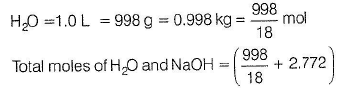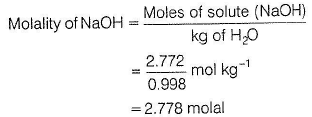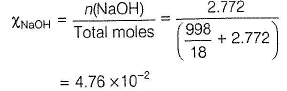QUESTION: 28

Comprehension Type

This section contains a passage describing theory, experiments, data, etc. Two questions related to the paragraph have been given. Each question has only one correct answer out of the given 4 options (a), (b), (c) and (d)

Passage

A handbook gives the concentration of water in a 2.772 M aqueous solution of NaOH as 998.0 g L -1.

Q.

Molality of solution is

Solution:

Molarity = 2.772 M =2.772 mol L-1  Thus, NaOH =2.772 molQUESTION: 29

A 5.2 molal aqueous solution of methyl alcohol (CH3OH) is supplied. What is the mole fraction of methyl alcohol in the solution?

Solution: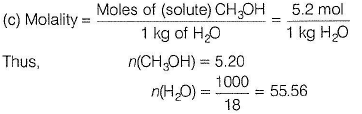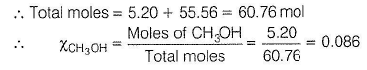*Answer can only contain numeric values
QUESTION: 30

One Integer Value Correct Type
This section contains 1 questions, when worked out will result in an integer value from 0 to 9 (both inclusive)

Q. Certain brine solution has 3.87% NaCI by mass with density 1.036 g mL-1. How many millilitre of this solution should be evaporated to obtain 0.32 g of NaCI?

Solution:

100 g brine solution has 3.87 g NaCI.